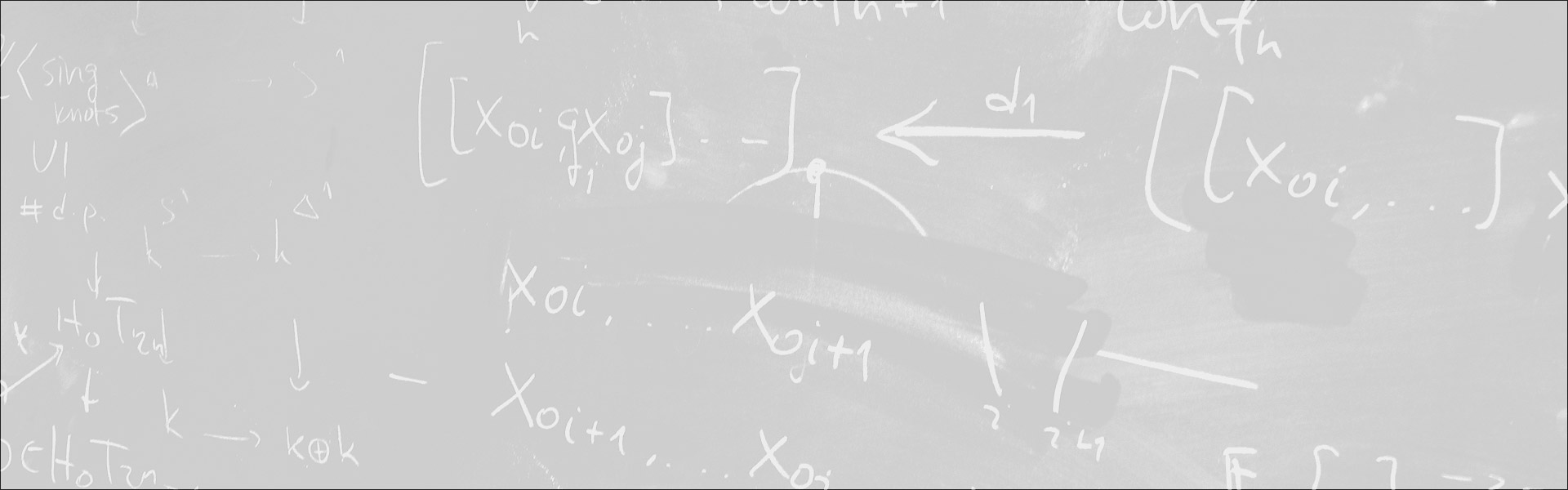Designed and built with care, filled with creative elements

Top# Curve-excluding fields

/  Évènements• Cet évènement est passé

## Curve-excluding fields

Let T be the theory of fields K of characteristic 0 such that the equation x^4 + y^4 = 1 has only four solutions in K. We show that T has a model companion. More generally, if K_0 is a field of characteristic 0 and C is a curve (affine or projective) of genus ≥ 2 with C(K_0) = &emptyset;, then there is a model companion CXF of the theory of fields K extending K_0 with C(K) = &emptyset;.
We can use this theory to construct a field K with an interesting combination of properties. On the model-theoretic side, the theory of K is complete, decidable, model-complete, and algebraically bounded, and K is a “geometric structure” in the sense of Hrushovski and Pillay. Additionally, some classification-theoretic properties might hold in K. On the field-theoretic side, K is non-large—there is a smooth curve C such that C(K) is finite and non-empty. This is unusual; the vast majority of model-theoretically tractable fields are large or finite. On the other hand, K is “virtually large”—it has a finite extension which is large. In fact, every proper algebraic extension of K is pseudo algebraically closed (PAC). The absolute Galois group of K is an ω-free profinite group. This negatively answers a question of Junker and Koenigsmann (is every model-complete infinite field large?) and a question of Macintyre (does every model-complete field have a small Galois group?).
This is based on joint work with Erik Walsberg and Vincent Ye.

- Séminaire Géométrie et théorie des modèles

#### Détails :

Orateur / Oratrice : Will Johnson
Date : 21 mai 2021
Horaire : 9h00 - 10h20
Lieu : Zoom